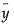The autocorrelation for the kth lag, computed as follows:
andis the mean of the N non-missing points in the time series. By definition, the first autocorrelation (lag 0) always has length 1.
The bars graphically depict the partial autocorrelations. The blue lines represent ± 2 standard errors for approximate 95% prediction limits, where the standard error is computed as follows:
The variogram measures the variance of the differences of points k lags apart and compares it to that for points one lag apart. The variogram is computed from the autocorrelations as follows:
where rk is the autocorrelation at lag k.

Help created on 9/19/2017